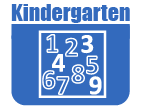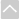# Math - KindergartenStand alone tasks are organized to support learning of content standards. These tasks can be used as initial instruction or to support students who are struggling with a particular topic.

Strand: MATHEMATICAL PRACTICES (K.MP)Strand: COUNTING AND CARDINALITY (K.CC)
Know number names and the counting sequence (Standards K.CC.1–3).
• Assessing Counting Sequences Part I
In this task the teacher asks the student to begin counting at a certain number and complete a sequence in order to assess their understanding of counting.
• Assessing Counting Sequences Part II
In this assessment the teacher gives the student a number and asks them to say which number comes after it.
This task is a one-on-one with a student where the teacher shows the numbers 1-10, one number at a time, in random order. The teacher asks, what number is this?"
• Assessing Sequencing Numbers
In this task the teacher asks student(s) to put numbers in order from the smallest number to the biggest number or in the order they would say them if they were counting.
• Assessing Writing Numbers
In this activity the teacher asks the student to write the number when spoken.
• More and Less Handfuls
Each student grabs two handfuls of counters. The student combines his/her handfuls into one collection and then counts them. The student then draws and records the quantity on a student-recording sheet.
Count to tell the number of objects (Standards K.CC. 4–5).
• Counting Mat
This task gives students another way to practice counting and gain fluency with connecting a written number with the act of counting. This task should be introduced by the teacher and would then be a good independent center.
• Counting Overview
This standard asks students to count with automaticity and meaning, and to be able to record their findings. Lastly, students need to be able to compare two numbers.
• Goodie Bags
In this task students compare 3 quantities and order them from least to greatest using various items.
• Goody Bags
In this activity students are given a bag of counting objects and they count the objects, record the number on a post-it note and stick the post-it note onto the outside of the bag.
• More and Less Handfuls
Each student grabs two handfuls of counters. The student combines his/her handfuls into one collection and then counts them. The student then draws and records the quantity on a student-recording sheet.
• The Napping House
The purpose of the task is for students to use the context of "The Napping House" to connect counting and cardinality. The teacher or students could also write a simple equation on the classroom dry-erase board each time another person or animal gets into the bed. This would connect counting to addition and subtraction for the students, and would connect with standard K.OA.1. For example, when the child gets in with granny, the equation would be 1+1=2 and so on for each animal added to the bed
Identify and compare quantities of objects and numerals (Standards K.CC.6–7).
• More and Less Handfuls
Each student grabs two handfuls of counters. The student combines his/her handfuls into one collection and then counts them. The student then draws and records the quantity on a student-recording sheet.
• Which number is greater? Which number is less? How do you know?
The purpose of this task is for students to explain how they know one quantity is greater or less than another quantity. Students will easily be able to identify which number is greater or less. However, explaining their reasoning will help them solidify their number sense skills.Strand: OPERATIONS AND ALGEBRAIC THINKING (K.OA)
Understand addition as putting together and adding to, and understand subtraction as taking apart and taking from (Standards K.OA.1–5).
• Bobbie Bear's Buttons
The purpose of this task is for students to find different pairs of numbers that sum to 4.
• Christina's Candies
This task is meant to be presented as a sequence of questions posed by the teacher to the students. Once a student finds one answer, the teacher then asks him/her to find another. The student is asked to use objects, pictures, or equations to demonstrate his/her thinking.
• IXL Game: Subtraction with Pictures - Numbers up to 10
This IXL game helps the kindergartener understand subtraction. This is just one of many online games that supports the Utah Math core. Note: The IXL site requires subscription for unlimited use.
• Make 9
In this task students are asked to make 9 in as many ways as they can by adding two numbers between 0 and 9.
• Many Ways to Do Addition 1
This lesson should be taught after students have had many experiences solving addition equations. The purpose of this task is not to teach or model the addition strategies. Rather the purpose of this task is make explicit different ways students can solve problems so that they will be able to find the most efficient strategy in any given situation and increase their addition fluency.
• My Book of Five
The purpose of this task is to help students develop fluency with addition facts to 5.Strand: NUMBER AND OPERATIONS IN BASE TEN (K.NBT)
Compose and decompose numbers 11–19 to gain foundations for place value (Standard K.NBT.1).
• What Makes a Teen Number?
The purpose of this task is to help students understand the base-ten structure of teen numbers. This task was designed specifically to support students in developing fluency with tens and teen numbers.Strand: MEASUREMENT AND DATA (K.MD)
Describe and compare measurable attributes of objects (Standards K.MD.1–2)
• Longer and Heavier? Shorter and Heavier?
The purpose of this task is for students to compare two objects according to different measurable attributes. Students should work on comparing objects along a single measurable attribute before tackling this task.
• Size Shuffle
The purpose of this task is for students to understand and practice using comparison language for height.
• Which is Heavier?
The teacher will pre-select a group of classroom objects (8-12) for the students to use. Each student will choose two objects to weigh. Each object will go in one side of the balance scale.
• Which is Longer?
The purpose of this task is for students to compare two objects to determine which object is longer and which object is shorter. This task asks students to work at the most basic level of measuring for kindergarteners, comparing one object to another without the use of a measuring tool.
Classify objects and count the number of objects in each category (Standard K.MD.3).
• Goodie Bags
In this task students compare 3 quantities and order them from least to greatest using various items.Strand: GEOMETRY (K.G)
Identify and describe shapes, including squares, circles, triangles, rectangles, hexagons, cubes, cones, cylinders, and spheres (Standards K.G.1–3).
Analyze, compare, create, and compose shapes (Standards K.G.4–6).The Online Core Resource pages are a collaborative project between the Utah State Board of Education and the Utah Education Network. If you would like to recommend a high quality resource, contact Trish French (Elementary) or Lindsey Henderson (Secondary). If you find inaccuracies or broken links contact resources@uen.org.